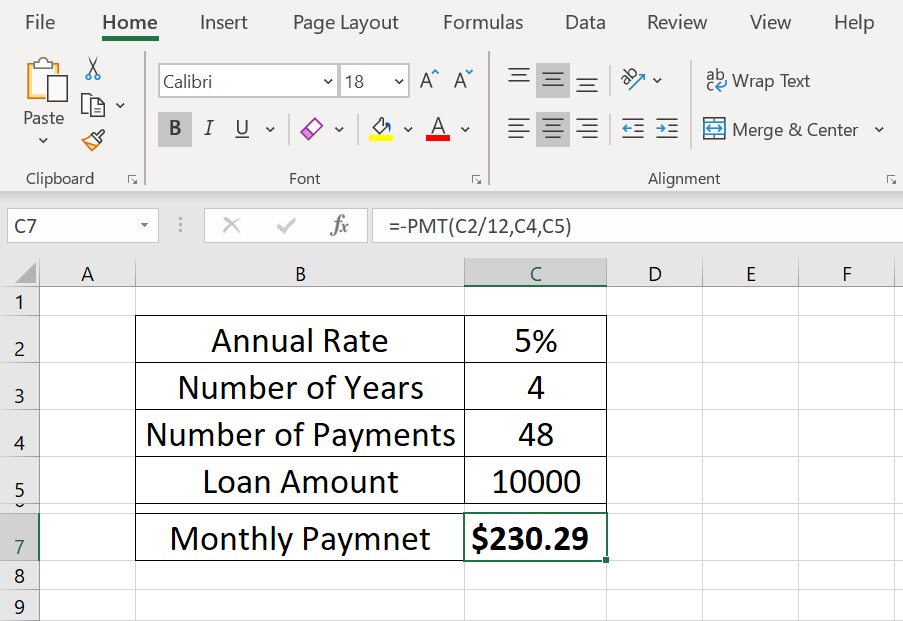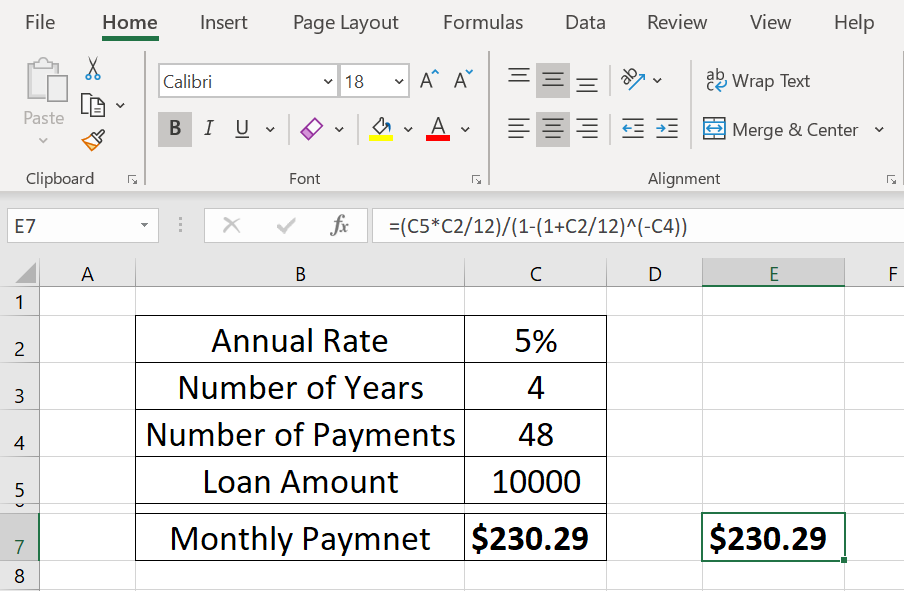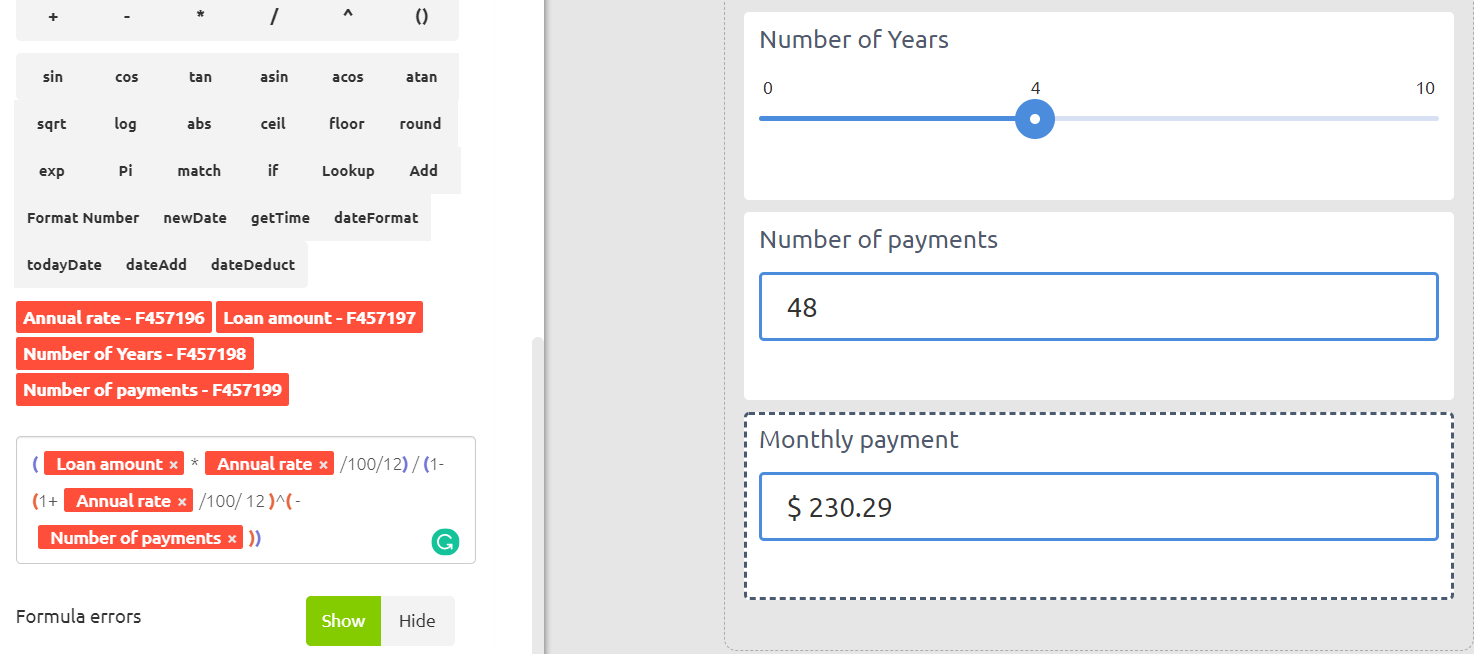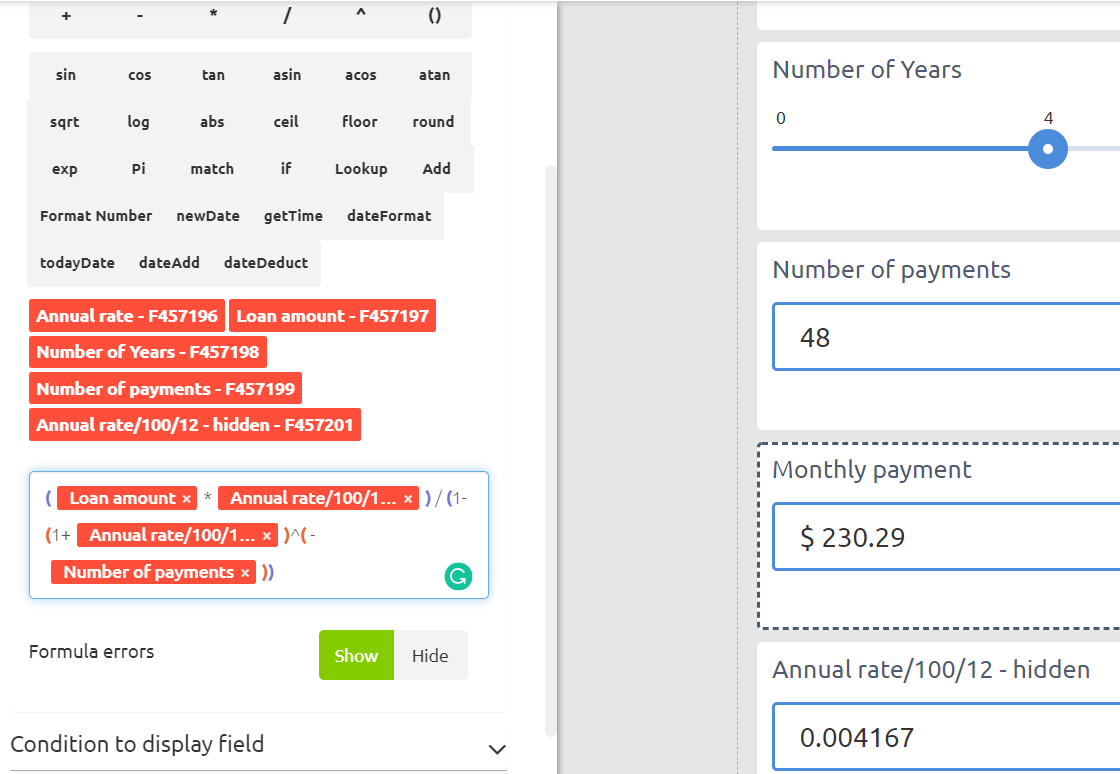Content

# How to present any Excel calculator in Calculoid – Web calculators case studies #8

With Calculoid you can make a web calculator from any of your Excel worksheets. In this case study, you will learn how to recreate your Excel calculations in Calculoid. It is much easier than it seems.

It is very simple to create a web-calculator based on a ready-to-use Excel file. Let's take a closer look at the example.

There is a financial function in Excel called PMT. It calculates monthly loan payments based on an annual rate, repayment period and loan amount. It is one of the most used Excel financial functions and a lot of our clients ask whether Calculoid can handle it. -Yes!

Example calculator in Excel:In Calculoid case studies #7 you can learn what are the main parts of web calculators and find multipurpose how-to flow describing the process of recreating web-form or Excel file in Calculoid.

Example calculator on the screenshot above has the following inputs:

• Annual rate (C2)
• Number of Years (C3)
• Loan amount (C5)

The result is:

• Monthly payment (C7)

Formulas and conditions are:

• PMT function in Monthly payment (C7).
• Number of payments (C4) - {Number of Years (C3) * 12}. The cell calculates the total number of payments based on the number of years entered (4), paid monthly (12).

First of all, we need to add all the fields to our calculator:

• Annual rate (C2) - Input field
• Number of Years (C3) - Slider field
• Number of payments (C4) - Formula field, hidden
• Loan amount (C5) - Input field
• Monthly payment (C7) - Formula field

Then we need to set the formulas. We know the formula for the Number of payments (C4). In Calculoid it will be Number of Years (Slider field) * 12. However, we don't know the formula for the PMT function. It is not presented in Excel. You can easily find it on the Internet or ask our This email address is being protected from spambots. You need JavaScript enabled to view it. to help.

This is the math formula for PMT function in Excel:

(C5*C2/12)/(1-(1+C2/12)^(-C4))Now we can add it to our calculator!Excel automatically converts 5% to 0.05 in calculations. In Calculoid you need to do it manually. That's why C2/12 in Excel formula equals to Annual rate/100/12 in our calculator.

It is nice to present Annual rate/100/12 formula in one hidden formula field. The new formula will be:There is always a way to improve your calculator. You can add more options to your calculator to compare paying loan weekly, fortnightly, or monthly. Let's add a Radio box field (How often?) to our calculator with three options:

• Weekly - 52 (option value)
• Fortnightly - 26 (option value)
• Monthly - 12 (option value)

Than formula in Annual rate/100/12 field is: Annual rate/100/How often?

The calculator has all the fields and formulas. Now, you can adjust the field sizes and change the layout of your calculator. Design settings will help you to style the calculator. Also, you can apply custom CSS styles in each field. Our team is always happy to assist you with custom design or fine-tune your calculator.

## Start Free Now

Create your own web calculator in a matter of minutes.
Test all features in the 30-day free trial.

No credit card or installation needed

### Information

info@calculoid.com

### Support

support@calculoid.com

### Easy Software Ltd.

Kemp House, 152-160